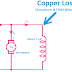We know that copper loss happens in both armature winding and field winding of a motor, whether it is DC or AC. Generally, it is more important for DC motors because it affects them more. But do you know what is copper loss? Let me tell you. When current flow through a conductive wire it is lightly opposed by its internal resistance. And when current is opposed by resistance for a time it will generate heat. This heat is useless. So as the electrical energy converts to useless heat energy it is a loss of energy. And it is known as copper loss. Copper loss is a variable loss because it depends upon the flow of current.

So the copper loss depends upon two parameters - current flow and resistance. If there is no current flow means no copper loss and if there is no resistance means no loss. But in practice, all the materials, objects, and conductors have minimum resistance.

## Copper Loss in Armature Winding

When current flows through the armature coil, some copper losses occur due to the internal resistance of the conductor of the armature coil. Generally, the armature coil for the motors is designed in such a way that it has a very low internal resistance so if the current flow is less then the copper loss also be very less. Copper loss in the armature coil is 30%-40% of total full load losses.

It can be measured by the following equation,

Ia2Ra

Here, Ia = Armature Current
Ra = Armature Resistance

Copper Loss Unit Watts.

As the armature has a fixed internal resistance, we can measure the estimated copper loss by the applied voltage. Here, a simple formula V=IR or I = V/R can be used. Here, V= Voltage across the armature coil, I = Current flow through the armature coil and R =  Armature resistance. So the final formula for the loss will be,   V2/Ra

So the copper loss is variable for the current flow in the armature current. If the variation in voltage changes the flow of current, the copper also is varied.

## Copper Loss in Field Winding

When current flows through the field winding, copper loss occurs due to the internal resistance of the field winding. In DC motors, field windings are connected in different ways such as Series, Shunt, etc. Generally, the filed copper loss is 20%-30% of the total full load losses.

### Series Field

When the field coil is connected in series with armature winding then it is called a Series Field Winding. Here, we cannot vary the current flow in the field winding separately.

Here the loss formula is,
Ise2Rse

Here, Ise = Series current through the field winding that is the same as armature current(Ia)
Rse = Resistance of the series field winding

So, here you may notice the copper loss in both the armature coil and series field coil will vary at the same time as the current flow through them is the same. But it does not mean that the loss in those both will be the same it depends upon their own internal resistance.

### Shunt Field

When the field winding is connected in parallel with the armature coil, it is called a shunt field winding. Here, we can vary the current flow through the field winding separately from the armature winding. The voltage across this winding is responsible for the current flow. Generally, the internal resistance of a shunt field winding is more than the series field winding.

Here, the loss formula is,
Ish2Rsh

Here, Ish = current flow through the shunt field winding that is different from the armature current
Rsh = internal resistance of the shunt field winding

Copper Loss in Armature and Field Winding of a MotorReviewed by Author on October 21, 2022 Rating: 5